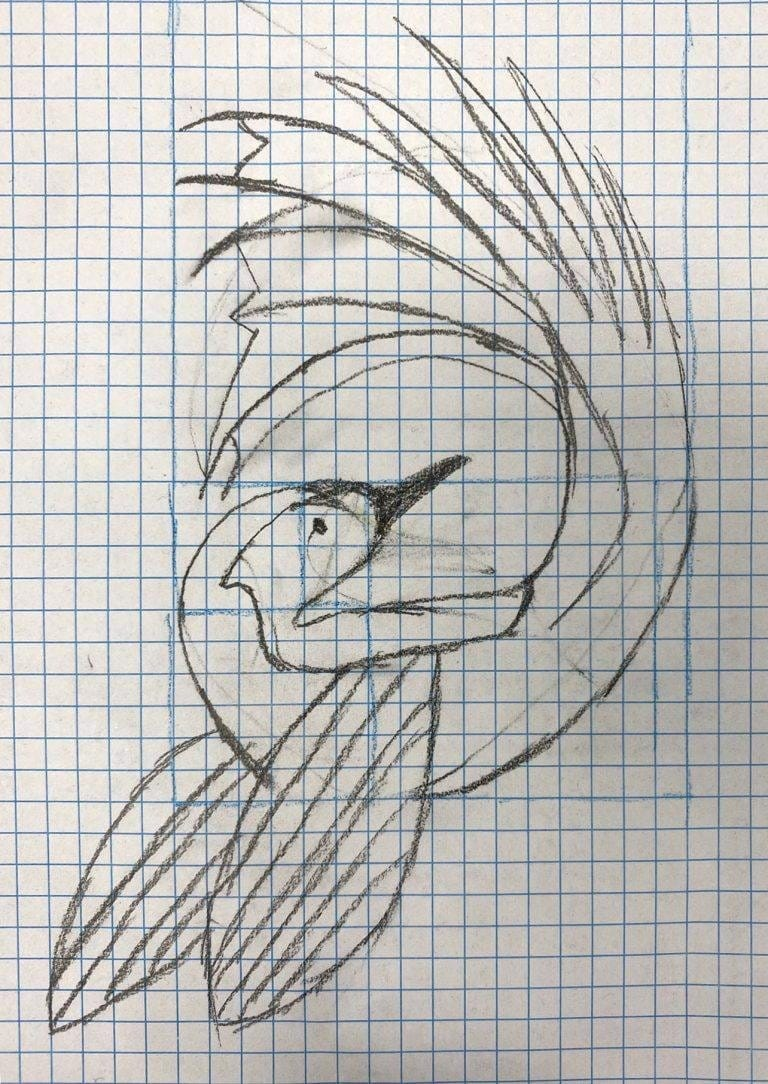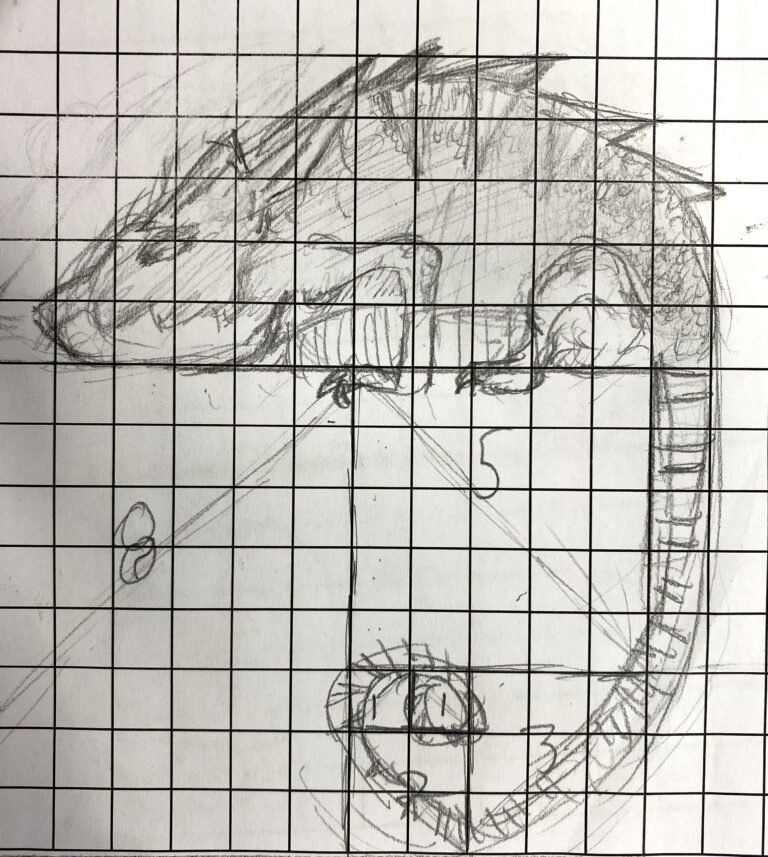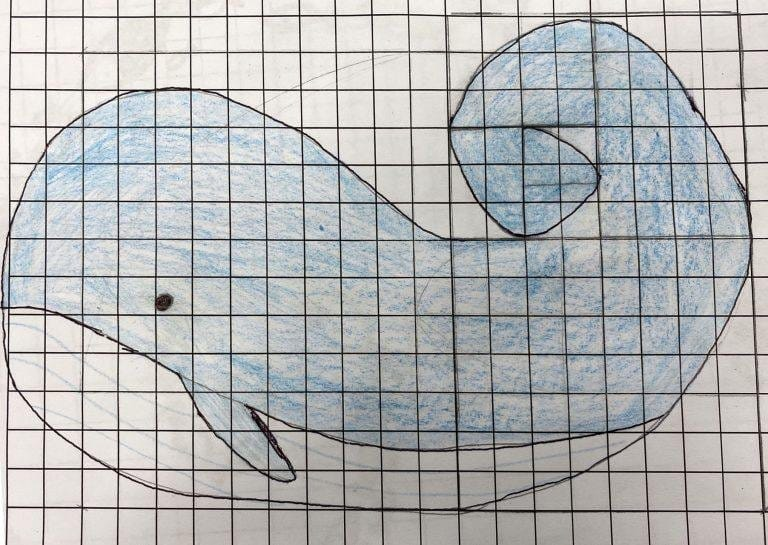# Golden rectangle

by Ginger Gyomber, Year 9

In Year 9 Maths, we have studied congruent and similar shapes. In this unit, we learnt about the golden rectangle. The term ‘golden rectangle’ refers to a rectangle that can be cut in two, to create a square and a rectangle that is similar to the original. The spiral of the golden rectangle is infinite. We looked at how the golden rectangle has influenced architecture and art over the years. We also learnt about the Fibonacci spiral. The Fibonacci spiral is like the golden rectangle, however it is solely made of squares and is finite. After researching these shapes, we were handed a piece of grid paper to make our own Fibonacci spiral. After measuring and drawing out the spiral, we were told to draw on it, creating an artwork inspired by the spiral.

Jasmine Ellis, Year 9

In Year 9 Maths class, we learnt about similar shapes and how they relate to the Golden rectangle and Fibonacci spirals. We looked at different Golden rectangles in famous architecture. Golden rectangle refers to a rectangle that can be cut into a square and another rectangle that is similar to the original. The Fibonacci spiral also known as the golden spiral is a sequence where the side length of the next square is equal to the sum of the previous two ones. By joining the corner with a curve, we were able to create a spiral. I measured out a rectangle and began to create a spiral. I then incorporated a whale into the drawing whose tail creates a Fibonacci spiral.